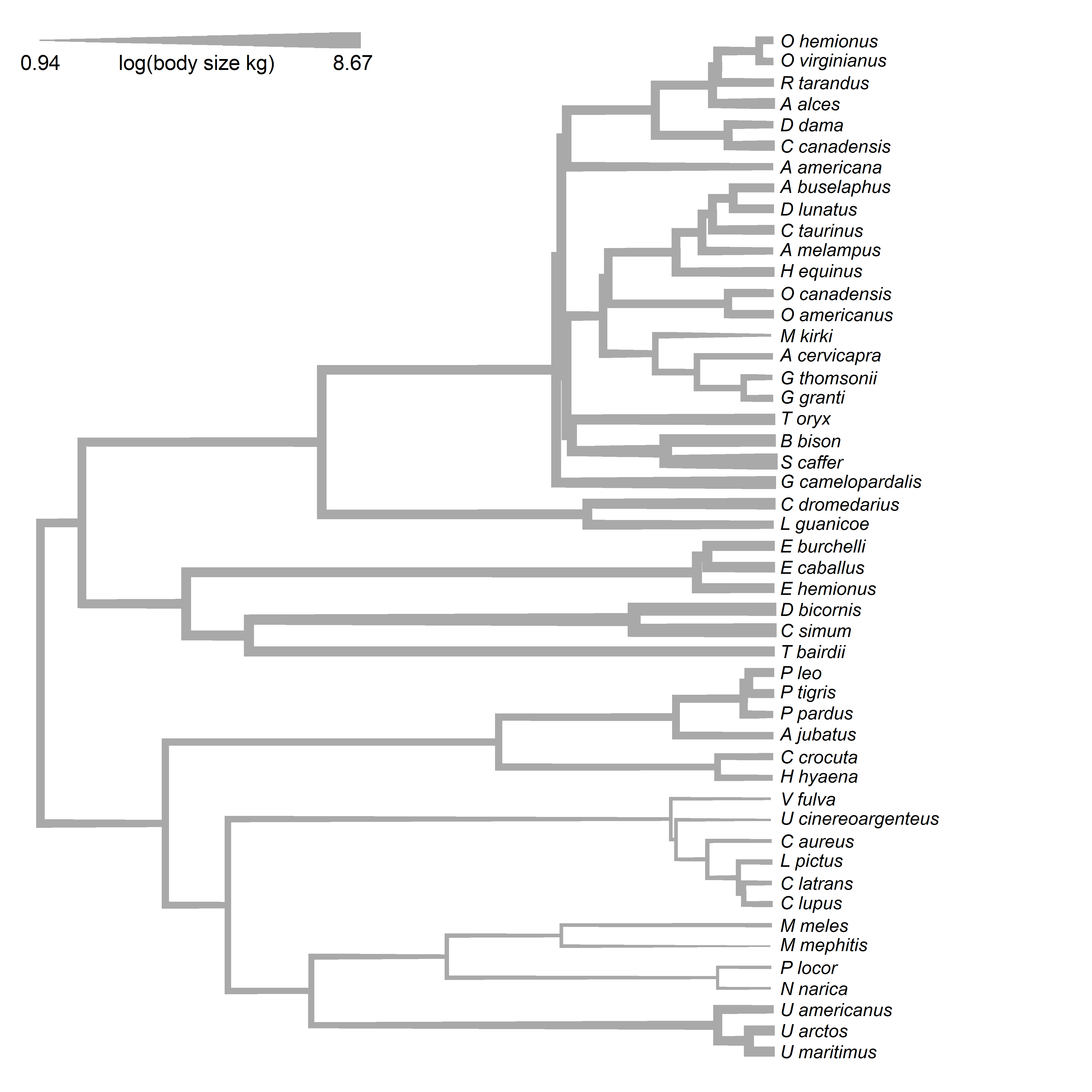## Thursday, July 16, 2020

### Mapping a continuous character on the tree using variable edge widths: Part II

Yesterday, in response to to a phytools user inquiry, I showed how to map a continuous character onto the tree using different edge widths. In my example, each individual branch width on the tree was determined by computing the average of the parent & daughter reconstructed or observed values for the trait. That is - every branch of the tree had a single width.

A little bit later, I realized that it would be nearly as easy to have branch width change as a (more or less - this all depends on how line widths are rendered by our plotting device) continuous function of the reconstructed trait value.

How I did this was first by adding a whole bunch of singleton (i.e., unbranching) nodes along all the edges of the tree. (I did this using phytools::make.era.map and phytools::map.to.singleton, but there are probably other ways of doing it.) Then, I reconstructed node states for all traits - both at branching & (all my new) unbranching nodes. Finally, I simply repeated the exercise of computing the trait value for all edges as the mean of the observed or estimated trait values for the nodes subtending that edge.

To get this to work, it is essential that you update phytools from GitHub. This is because I recently pushed an update to fastAnc that allows it to work with trees containing unbranching nodes. The easiest way to update phytools from GitHub is by using devtools as follows:

library(devtools)
install_github("liamrevell/phytools")


Here's how it works using the same mammal dataset as yesterday.

library(phytools)
data(mammal.tree)
data(mammal.data)
lnBodyMass<-setNames(log(mammal.data$bodyMass), rownames(mammal.data)) h<-max(nodeHeights(mammal.tree)) ## this is the number of unbranching nodes ## that I'm going to add along any path from ## the root to any tip n<-100 LL<-setNames(seq(0,h,length.out=n),1:n) tmp<-make.era.map(mammal.tree,LL) mammal.tree<-map.to.singleton(tmp) a<-fastAnc(mammal.tree,lnBodyMass) node.values<-c(lnBodyMass[mammal.tree$tip.label],a)
edge.values<-apply(mammal.tree$edge,1,function(e,nv) mean(nv[e]),nv=node.values) edge.widths<-edge.values-min(edge.values) edge.widths<-edge.widths*(9/max(edge.widths))+1 par(lend=2) plot(mammal.tree,no.margin=TRUE,cex=0.7,edge.width=edge.widths, label.offset=1,edge.col="darkgrey") leg.length<-30 nsegs<-20 segments(x0=seq(0,(1-1/nsegs)*leg.length,length.out=nsegs), y0=rep(Ntip(mammal.tree),nsegs), x1=seq(1/nsegs*leg.length,leg.length,length.out=nsegs), y1=rep(Ntip(mammal.tree),nsegs), lwd=seq(1,10,length.out=nsegs),col="darkgrey") text(0,Ntip(mammal.tree),round(min(edge.values),2),pos=1, cex=0.8) text(leg.length,Ntip(mammal.tree),round(max(edge.values),2), pos=1,cex=0.8) text(leg.length/2,Ntip(mammal.tree),"log(body size kg)", pos=1,cex=0.8)OK, that's pretty cool. Next, let's try it with a different dataset. data(sunfish.tree) data(sunfish.data) gape.width<-setNames(sunfish.data$gape.width,
rownames(sunfish.data))
h<-max(nodeHeights(sunfish.tree))
n<-100
LL<-setNames(seq(0,h,length.out=n),1:n)
tmp<-make.era.map(as.phylo(sunfish.tree),
LL)
sunfish.tree<-map.to.singleton(tmp)
a<-fastAnc(sunfish.tree,gape.width)
node.values<-c(gape.width[sunfish.tree$tip.label],a) edge.values<-apply(sunfish.tree$edge,1,function(e,nv)
mean(nv[e]),nv=node.values)
edge.widths<-edge.values-min(edge.values)
max.width<-15
edge.widths<-edge.widths*(max.width/max(edge.widths))+1
par(lend=2)
plot(sunfish.tree,no.margin=TRUE,edge.width=edge.widths,
label.offset=0.01,edge.col=palette(),
x.lim=c(0,0.27),y.lim=c(1,Ntip(sunfish.tree)+1))
leg.length<-0.45*h
nsegs<-30
segments(x0=seq(0,(1-1/nsegs)*leg.length,length.out=nsegs),
y0=rep(Ntip(sunfish.tree)+1,nsegs),
x1=seq(1/nsegs*leg.length,leg.length,length.out=nsegs),
y1=rep(Ntip(sunfish.tree)+1,nsegs),
lwd=seq(1,max.width,length.out=nsegs),col=palette())
text(0,Ntip(sunfish.tree)+0.75,round(min(edge.values),2),pos=1,
cex=0.8)
text(leg.length,Ntip(sunfish.tree)+0.75,round(max(edge.values),2),
pos=1,cex=0.8)
text(leg.length/2,Ntip(sunfish.tree)+0.75,"relative gape width",
pos=1,cex=0.8)